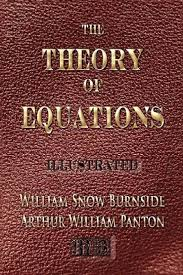### Theory of Equations

Course Overview:

Theory of Equations is one of the elementary courses in mathematics. The students will be learning about polynomials, equations of degree “n”, techniques to solve equations, to graph etc. for given polynomial. This course knowledge is of particular relevance in situations where

·         Mathematical modelling is involved.

·         Visual presentation and data interpretation is crucial.

·         Some trends are observed and etc.

Course Outcomes

The student completing this course,

CO-1                     Is equipped with various techniques to solve equations of degree ≤ 4.

CO-2                     Will be able to perform transformation of equations.

CO-3                     Should be able to use the GeoGebra software for graphing various polynomials.

CO-4                     Becomes conceptually strong to grasp the geometrical ideas during further studies.

CO-5                     Will be able to interpret the solutions of equations as necessary.

CO-6                     Should be able to try mathematical modelling of some meaningful real world problems.